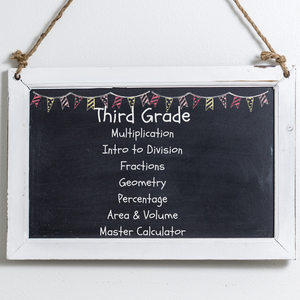# Club Sage (Age 8-9)

Regular price
\$59.95
Sale price
\$59.95
Regular price
Sold out
Unit price
per

We start with large operations and make is easy and straight forward.  We allow for ample practice on specific topics like multiplication and division before we level up to fractions.

Our section of fractions is the funnest.  It's easy, fun and understanding the concepts.

WHAT THEY LEARN

Operations and Algebraic Thinking

• Understanding Multiplication with pictures
• Quotients
• Problem Solving with Multiplication and Missing Factors
• Problem Solving with Division
• Problem Solving with Division and Three Digit Numbers
• Multiplication and Division with an unknown number
• Properties of Multiplication
• Fact Families with Multiplication and Division
• Understanding Division
• Multiplication Chart
• Division Chart
• Multi-step Word Problems
• Using Variable Equations to solve word problems
• Rounding
• Estimation to solve word problems
• Addition, Subtraction, Multiplication and Division pattern tables

Numbers and Operations in Base 10:

• Place Value with Rounding
• Adding and Subtracting with 1000
• Word Problem
• Multiplying one digit numbers by multiples of 10

Geometry:

• Solid Figures
• Plane Shapes
• Equal Parts

Measurement and Data:

• Telling Time
• Elapsed Time
• Time Word Problems
• Time Lines
• Metric Measurement and Word Problem
• Bar Graphs
• Pictographs
• Measuring and Line Plots
• Area and Square Units
• Area of a rectangle
• Area word problems
• Perimeter
• Area v. Perimeter word problem

Fractions:

• Identifying Fractions
• Fractions on a Number line
• Equivalent Fractions
• Reducing Fractions
• Ordering Fractions
• Whole Numbers and Fractions
• Mixed Numbers
• Comparing Fractions
• Fraction Word Problems